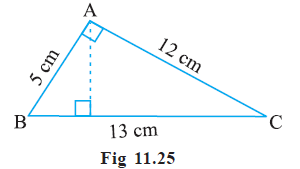# Ex.11.2 Q7 Perimeter and Area - NCERT Maths Class 7

Go back to  'Ex.11.2'

## Question

$$\triangle ABC$$ is right angled at$$\, A \,$$ (Fig 11.25).  $$AD$$ is perpendicular to $$BC$$. If $$AB = 5 \,\rm cm$$, $$BC = 13 \,\rm cm$$ and $$AC = 12 \,\rm cm$$, Find the area of $$\triangle ABC$$. Also find the length of $$AD$$.Video Solution
Perimeter And Area
Ex 11.2 | Question 7

## Text Solution

What is known?

$$\triangle ABC$$ is right angled at $$A$$, $$AD$$ is perpendicular to side $$BC$$. Length of three sides of the triangle is also given.

What is unknown?

The area of $$\triangle ABC$$ and the length of perpendicular $$AD$$.

Reasoning:

First, find the area of triangle using base $$(5 \,\rm cm)$$ and height $$(12 \,\rm cm)$$. Now, the area of the triangle is known, and the base is given as $$BC = 13 \,\rm cm$$ and you must find the height $$AD$$ by using the formula of area of triangle.

Steps:

In right angled $$\triangle BAC$$,

$$AB = 5 \,\rm cm$$, $$BC = 13 \,\rm cm$$ and $$AC = 12 \,\rm cm$$.

Area of triangle $$BAC$$  $$=$$ $$\frac{1}{2}$$ Base  $$\times$$ Height

\begin{align}&= \frac{1}{2} \times 5 \times 12\\&= 30\;\rm{c{m^2}}\end{align}

Area of triangle $$ABC$$  $$=$$ $$\frac{1}{2}$$ Base  $$\times$$ Height

\begin{align}30 &= \frac{1}{2} \times 13 \times {\rm{AD}}\\{\rm{AD}} &= \frac{{30 \times 2}}{{13}}\\{\rm{AD}} &= \frac{{60}}{{13}}\\{\rm{AD}} &= 4.61\;cm\end{align}

Learn from the best math teachers and top your exams

• Live one on one classroom and doubt clearing
• Practice worksheets in and after class for conceptual clarity
• Personalized curriculum to keep up with school